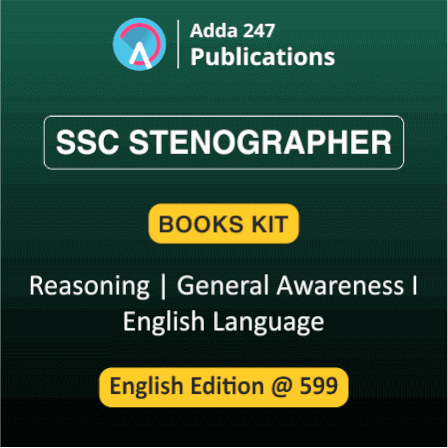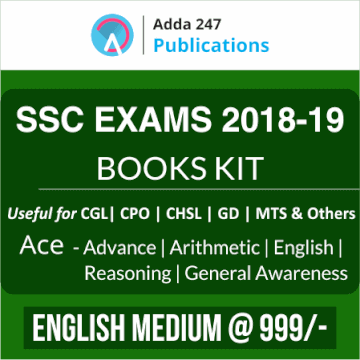# Reasoning Questions for SSC and Railway Exam 2018: 24th December

Dear Students,

As you all know, the upcoming months are lined up with various important exams like SSC Translator, SSC Stenographer, RRB ALP, RPF SI and constable and many more, so we are here to help you with the subject that is common to all of the given exams. We are providing daily reasoning quizzes, practicing which will help you to score good marks in this section. We aim to provide the best study material to our readers with exam level questions to help them get used to the recent pattern. Attempt this quiz and check your preparation.

Q1. Select the related word/letters/numbers from the given alternatives.
Accommodation : Rent : : Journey : ?
(a) Expense
(b) Octroi
(c) Freight
(d) Fare

Q2.  For the following question, find the odd word/letters/number from the given alternatives.
(a) Kilogram
(b) Quintal
(c) Ton
(d) Gallon

Q3. A series is given, with one term missing. Choose the correct alternative from the given ones that will complete the series.
ZXV, USQ, PNL, ?
(a) MJH
(b) JHF
(c) KIG
(d) KJI

Q4. A gets more marks than B but less than C. D gets less marks than E but more than A. If C gets less than D then who amongst A, B, C, D, E gets the highest marks?
(a) C
(b) D
(c) E
(d) B

Q5. From the given alternative words, select the word which cannot be formed using the letters of the given word.
EXORBITANT
(a) BITTER
(b) EXPORT
(c) EXNORA
(d) EXTANT

Q6. If D = 4
DESK = 39, then the value of DRAW is
(a) 57
(b) 46
(c) 45
(d) 36

Q7. If ‘÷’ stands for subtraction, ‘–’ stands for addition, ‘×’ stands for division and ‘+’ stands for multiplication, then which one of the following equation is correct?
(a) 35 ÷ 4 – 25 × 5 + 5 = 28
(b) 35 ÷ 4 – 25 × 5 + 5 = 61
(c) 35 ÷ 4 – 25 × 5 + 5 = 41
(d) 35 ÷ 4 – 25 × 5 + 5 = 56

Q8. In this question, some equations are solved on the basis of a certain system. On the same basis find out the correct answer from amongst the four alternatives for the unsolved equation.
15 × 26 = 6512
29 × 36 = 6923,
46 × 54 = ?
(a) 5464
(b) 4645
(c) 4564
(d) 4465

Q9. Select the missing numbers from the given alternatives.

(a) 19
(b) 16
(c) 21
(d) 20

Q10. Rohan walks a distance of 3 kms towards North, then turns to his left and walks for 2 kms. He again turns left and walks for 3kms. At this point he turns to his left and walks for 3 kms. How far is he from the starting point?
(a) 5 kms
(b) 3 kms
(c) 2 kms
(d) 1 km

Q11. Identify the diagram that best represents the relationship among the classes given below.
Criminals, Judges, Thieves

(a) A
(b) B
(c) C
(d) D

Q12. Which answer figure will complete the pattern in the question figure?

(a) A
(b) B
(c) C
(d) D

Q13. For the following question, find the odd word/letters/number from the given alternatives.
(a) 36
(b) 64
(c) 54
(d) 108

Q14. In the following question, one term in the number series is wrong. Find out the wrong term.
3, 10, 27, 4, 16, 64, 5, 25, 12
(a) 3
(b) 4
(c) 10
(d) 27

Q15. Matrices can be represented first by its row and next by its column, e.g., ‘L’ can be represented by 00, 13, 24 etc., and ‘T’ can be represented by 59, 66, 78, etc.
Similarly you have to identify the set for the word ‘CORK’

(a) 42, 21, 67, 58
(b) 23, 33, 86, 85
(c) 11, 40, 55, 75
(d) 30, 14, 97, 56

You may also like to read: